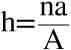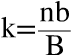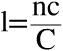# Summary: Materials Science And Engineering | 9781118319222 | William D Callister, et al• This + 400k other summaries
• A unique study and practice tool
• Never study anything twice again
• Get the grades you hope for
• 100% sure, 100% understanding
Remember faster, study better. Scientifically proven.## Read the summary and the most important questions on Materials Science and Engineering | 9781118319222 | William D. Callister; David G. Rethwisch

• ### 3 Fundamentals of Crystallography

This is a preview. There are 2 more flashcards available for chapter 3

• ### 3.2 Fundamental Concepts

This is a preview. There are 5 more flashcards available for chapter 3.2

• #### What is the atomic hard-sphere model?

In the atomic hard-sphere model, atoms are represented as solid spheres. In this model nearest-neighbor atoms touch each other.
• #### What does the term lattice mean in context of crystal structures?

Here lattice means a three-dimensional array of points coinciding with atom position.
• ### 3.3 Unit Cells

This is a preview. There are 1 more flashcards available for chapter 3.3

• #### Why is it convenient to use unit cells?

Since atoms occur in a repetitive array in crystal structures, it is possible to subdivide the structure, resulting in a parallelepiped.
• ### 3.4 Crystal Systems

This is a preview. There are 14 more flashcards available for chapter 3.4

• #### Wat zijn de roosterparameters (lattice paramters)

X,y,z = coördinaat assen
a,b,c= axiale lengte
a,B,y= interaxiale hoeken
• #### What are the axial relationships and interaxial angles of the Hexagonal crystal system?

Axial relationship: a = b ≠ c
Interaxial angles: α = β = 90° ,γ = 120°
• ### 3.5 Point Coordinates

This is a preview. There are 1 more flashcards available for chapter 3.5

• #### How are the point coordinate indices named and calculated?

q, r and s
qa = lattice position referenced to the x axis
rb = lattice position referenced to the y axis
sc = lattice position referenced to the z axis
• ### 3.6 Crystallographic Directions

This is a preview. There are 5 more flashcards available for chapter 3.6

• #### What is the Miller-Bravais coordinate system?

It is a four-axis coordinate system used for hexagonal crystals.
• ### 3.7 Crystsallographic Planes

This is a preview. There are 2 more flashcards available for chapter 3.7

• #### How do you determine de Miller indices (hkl)?

1. Make sure the plane does not pass trough the origin O.
2. Find the coordinates of the intersection between the plane and the x, y and z axes these will be designated A, B and C.
3. Calculate the reciprocal and normalize A, B and C.
4. Multiply or divide by a common factor to get the smallest integer.
5. In summary:,,• ### 3.8 Single Crystals

• #### What is the definition of a single crystal?

When the unit cell is periodically repeated in the entire crystal, it is called a single crystal.
• ### 3.9 Polycrystallinge Materials

• #### What is meant by grains and grain boundaries?

A grain is a small crystal within a crystalline solid. The area where grains meet and where there is an atomic mismatch is called the grain boundary.